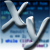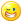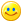## Welcome to the Second Life Forums Archive

These forums are CLOSED. Please visit the new forums HERE

# Simplified llAxes2Rot interface

Xylor Baysklef
Scripting AddictJoin date: 4 May 2003
Posts: 109
08-22-2003 00:56
This is an alternate method for using llAxes2Rot... it only uses two axes for the call, and the parameters are taken as strings. I use strings here, because I usually get the orientation of an object from a notecard or constants, and having the user type in "x" or "-y" seems simpler then typing <1, 0, 0> or <0, -1, 0>.

The two parameters it takes are Forward and Up, which are the direction the object's forward and up axes point. Just have the user select the object, turn on "Local Axis", and look at which axes are pointing in those two directions.

CODE
`vector StringToVector(string axis) {    string AxisDir;    string AxisName;        // Check the string length; they may have omitted the '+'.    if (llStringLength(axis) == 1) {        AxisDir = "+";        AxisName = llToLower( axis );    }    // Otherwise lets split up the string.    else {        AxisDir  = llGetSubString(axis, 0, 0);        AxisName = llToLower( llGetSubString(axis, 1, 1) );    }        vector Vec;    if (AxisName == "x")        Vec = <1, 0, 0>;            if (AxisName == "y")        Vec = <0, 1, 0>;            if (AxisName == "z")        Vec = <0, 0, 1>;            if (AxisDir == "-")        Vec *= -1.0;            return Vec;}vector CrossProduct(vector a, vector b) {    // Compute the cross product.    return <a.y * b.z - a.z * b.y,            a.z * b.x - a.x * b.z,            a.x * b.y - a.y * b.x>;}rotation AxesToRotation(string fwd_dir, string up_dir) {    // Convert from strings to vectors.    vector Fwd  = StringToVector(fwd_dir);    vector Up   = StringToVector(up_dir);    // Fill in the missing axis.    vector Left = llVecNorm( CrossProduct(Up, Fwd) );        // If Left is ZERO_VECTOR, then Fwd and Up are parellel.    if (Left == ZERO_VECTOR) {        llSay(0, "ERROR: Forward and Up vectors are parallel!");    }    rotation Rot = llAxes2Rot(Fwd, Left, Up);    // Return the rotation going in the opposite direction.    Rot.s = -Rot.s;    return Rot;}`

I use these functions in various scripts where the orientation of the object is important (vehicles, follower pets, etc). Also, I am using the opposite rotation returned by llAxes2Rot, as I find it more useful in my applications (ie, llSetRot(AxesToRotation("-x", "y"); could be used directly, without fiddling with the rotation).

Xylor
Tiger Crossing
The Prim MakerJoin date: 18 Aug 2003
Posts: 1,560
08-25-2003 19:36
Cool that you wrote a cross product function, but it's also built into LSL with the % operator.~ Tiger Crossing
~ (Nonsanity)
Xylor Baysklef
Scripting AddictJoin date: 4 May 2003
Posts: 109
08-27-2003 08:03
Thanks Tiger =). I should have looked harder for it, since it goes hand in hand with vectors =/.

Xylor